# Optical solitons

lect10.pdf [496 kB] Lecture 10 in Portable Document Format.

### Contents

In this lecture, we will focus on examples of electromagnetic wave propagation in optical Kerr media (that is to say, essentially with a refractive index depending on the optical intensity), by applying the forms of Maxwell's equations as obtained in the eighth lecture. In particular, we will show how optical pulses may experience a self-modulation compensating for the naturally occurring dispersion, to yield so-called solitons which can propagate over very long distances without changing shape.

The outline for this lecture is:

• What are solitons?
• Basics of soliton theory
• Spatial and temporal solitons
• The mathematical equivalence between spatial and temporal solitons
• The creation of temporal and spatial solitons

## 1. What are solitons?

The first reported observation of solitons was made in 1834 by John Scott Russell, a Scottish scientist and later famous Victorian engineer and shipbuilder, while studying water waves in the Glasgow-Edinburgh channel. As part of this investigation, he was observing a boat being pulled along, rapidly, by a pair of horses. For some reason, the horses stopped the boat rather suddenly, and the stopping of the boat caused a verystrong wave to be generated. This wave, in fact, a significant hump of water stretching across the rather narrow canal, rose up at the front of the boat and proceeded to travel, quite rapidly down the canal. Russell, immediately, realised that the wave was something very special. It was "alone", in the sense that it sat on the canal with no disturbance to the front or the rear, nor did it die away until he had followed it for quite a long way. The word "alone" is synonymous with "solitary", and Russell soon referred to his observation as the Great Solitary Wave.

The word "solitary" is now routinely used, indeed even the word "solitary" tends to be replaced by the more generic word "soliton". Once the physics behind Russell's wave is understood, however, solitons, of one kind or another, appear to be everywhere but it is interesting that the underlying causes of soliton generation were not understood by Russell, and only partially by his contemporaries.

## 2. Classes of solitons

### 2.1. Bright temporal envelope solitons

Pulses of light with a certain shape and energy that can propagate unchanged over large distances. This is the class of solitons which we will focus on in this lecture.

### 2.2. Dark temporal envelope solitons

Pulses of "darkness" within a continuous wave, where the pulses are of a certain shape, and possess propagation properties similar to the bright solitons.

### 2.3. Spatial solitons

Continuous wave beams or pulses, with a transverse extent of the beam that via the refractive index change due to optical Kerr-effect can compensate for the diffraction of the beam. The optically induced change of refractive index works as an effective waveguide for the light.

## 3. The normalized nonlinear Schrödinger equation for temporal solitons

The starting point for the analysis of temporal solitons is the time-dependent wave equation for the spatial envelopes of the electromagnetic fields in optical Kerr-media, here for simplicity taken for linearly polarized light in isotropic media,(1)

where, as previously, vg = (dk/)-1 is the linear group velocity, and where we introduced the notationfor the second order linear dispersion of the medium, and (in analogy with Butcher and Cotter Eq.~(6.63)),for the intensity-dependent refractive index $n=n_0+n_2|{\bf E}_{\omega}|^2$. Since we here are considering wave propagation in isotropic media, with linearly polarized light (for which no polarization state cross-talk occur), the wave equation~(1) is conveniently taken in a scalar form as(2)

Equation (2) consists of three terms that interact. The first two terms contain first order derivatives of the envelope, and these terms can be seen as the homogeneous part of a wave equation for the envelope, giving travelling wave solutions that depend on the other two terms, which rather act like source terms.

The third term contains a second order derivative of the envelope, and this terms is also linearly dependent on the dispersion $\beta$ of the medium, that is to say, the change of the group velocity of the medium with respect to the angular frequency $\omega$ of the light. This term is generally responsible for smearing out a short pulse as it traverses a dispersive medium.

Finally, the fourth term is a nonlinear source term, which depending on the sign of $n_2$ will concentrate higher frequency components either at the leading or trailing edge of the pulse, as soon will be shown.

### 3.1. The effect of dispersion

The group velocity dispersion d vg/dω is related to the introduced dispersion parameter β = d2k/dω2 asand hence the sign of the group velocity dispersion is the opposite of the sign of the dispersion parameter β. In order to get a qualitative picture of the effect of linear dispersion, let us consider the effect of the sign of β:

#### 3.1.1. β > 0, negative group velocity dispersion

For this case, the group velocity dispersion is negative, sinceThis implies that the group velocity decreases with an increasing angular frequency ω. In other words, the "blue" frequency components of the pulse travel slower than the "red" components. Considering the effects on the pulse as it propagates, the leading edge of the pulse will after some distance contain a higher concentration of low ("red") frequencies, while the trailing edge rather will contain a higher concentration of high ("blue") frequencies. This effect is illustrated in Fig. 1.Whenever red'' frequency components travel faster than blue'' components, we usually associate this with so-called normal dispersion.

#### 3.1.2. β < 0, positive group velocity dispersion

For this case, the group velocity dispersion is instead positive, since nowThis implies that the group velocity increases with an increasing angular frequency ω. In other words, the "blue" frequency components of the pulse now travel faster than the "red" components. Considering the effects on the pulse as it propagates, the leading edge of the pulse will after some distance hence contain a higher concentration of high ("blue") frequencies, while the trailing edge rather will contain a higher concentration of low ("red") frequencies. This effect, being the inverse of the one described for a negative group velocity dispersion, is illustrated in Fig.~2.Whenever "blue" frequency components travel faster than "red" components, we usually associate this with so-called anomalous dispersion.

Notice that depending on the distribution of the frequency components of the pulse as it enters a dispersive medium, the pulse may for some propagation distance actually undergo pulse compression. For β > 0, this occurs if the leading edge of the pulse contain a higher concentration of "blue" frequencies, while for β < 0, this occurs if the leading edge of the pulse instead contain a higher concentration of "red" frequencies.

### 3.2. The effect of a nonlinear refractive index

Having sorted out the effects of the sign of β on the pulse propagation, we will now focus on the effects of a nonlinear, optical field dependent refractive index of the medium.

In order to extract the effect of the nonlinear refractive index, we will here go to the very definition of the instantaneous angular frequency of the light from its real-valued electric field,For light propagating in a medium where the refractive index depend on the intensity as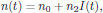the spatial envelope will typically be described by an effective propagation constant (see lecture notes as handed out during lecture nine)and the local, instantaneous angular frequency becomesThe typical behaviour of the instantaneous angular frequancy ωloc(t) on a typical pulse shape is shown in Fig. 3, for the case of n2 > 0 and a Gaussian pulse.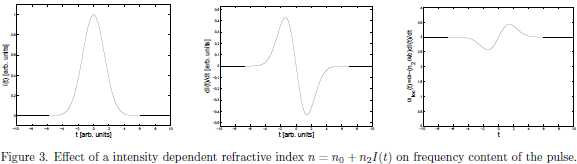As seen in the figure, the leading edge of the pulse has a slight decrease in angular frequency, while the trailing edge has a slight increase. This means that in the presence of an intensity dependent refractive index, for n2 > 0, the pulse will have a concentration of "red" frequencies at the leading edge, while the trailing edge will have a concentration of "blue" frequencies. This is illustrated in Fig. 4.If instead n2 < 0, that is to say, for an intensity dependent refractive index that decrease with an increasing intensity, the roles of the "red" and "blue" edges of the pulse are reversed.

### 3.3. The basic idea behind temporal solitons

As seen from Figs. 2 and 4, the effect of anomalous dispersion (with β < 0) and the effect of a nonlinear, intensity dependent refractive index (with n2 > 0) are opposite of each other. When combined, that is to say, considering pulse propagation in a medium which simultaneously possesses anomalous dispersion and n2 > 0, these effects can combine, giving a pulse that can propagate without altering its shape. This is the basic principle of the temporal soliton.

### 3.4. Normalization of the nonlinear Schrödinger equation

Equation (2) can now be cast into a normalized form, the so-called nonlinear Schrödinger equation, by applying the change of variables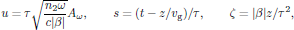where τ is some characteristic time of the evolution of the pulse, usually taken as the pulse duration time, which gives the normalized form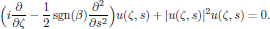(3)

This normalized equation has many interesting properties, and for some cases even analytical solutions exist, as we will see in the following sections. Before actually solving the equation, however, we will consider another mechanism for the generation of solitons.

Before leaving the temporal pulse propagation, a few remarks on the signs of the dispersion term β and the nonlinear refractive index n2 should be made. Whenever β > 0, the group velocity dispersionwill be negative, and the pulse will experience what we call a normal dispersion, for which the refractive index of the medium decrease with an increasing wavelength of the light. This is the "common" way dispersion enters in optical processes, where the pulse is broadened as it traverses the medium.

## 4. Spatial solitons

As a light beam with some limited spatial extent in the transverse direction enter an optical Kerr media, the intensity variation across the beam will via the intensity dependent refractive index n = n0+n2I form a lensing through the medium. Depending on the sign of the coefficient n2 (the "nonlinear refractive index"), the beam will either experience a defocusing lensing effect (if n2 < 0) or a focusing lensing effect (if n2 > 0); in the latter case the beam itself will create a self-induced waveguide in the medium (see Fig. 5).As being the most important case for beams with maximum intensity in the middle of the beam (as we usually encounter them in most situations), we will focus on the case n2 > 0. For this case, highly intense beams may cause such a strong focusing that the beam eventually break up again, due to strong diffraction effects for very narrow beams, or even due to material damage in the nonlinear crystal.

For some situations, however, there exist stationary solutions to the spatial light distribution that exactly balance between the self-focusing and the diffraction of the beam. We can picture this as a balance between two lensing effects, with the first one due to self-focusing, with an effective focal length $f_{\rm foc}$ (see Fig.~6), and the second one due to diffraction, with an effective focal length of $f_{\rm defoc}$ (see Fig. 7).Whenever these effects balance each other, we in this picture have the effective focal length ffoc+fdefoc = 0.

In the electromagnetic wave picture, the propagation of an optical continuous wave in optical Kerr-media is governed by the wave equation(4)

with k = ωn0/c, using notations as previously introduced in this course. For simplicity we will from now on consider the spatial extent of the beam in only one transverse Cartesian coordinate x.

By introducing the spatial envelope Aω(x,z) according toand using the slowly varying envelope approximation in the direction of propagation z, the wave equation (4) takes the form(5)

Notice the strong similarity between this equation for continuous wave propagation and the equation (3)for the envelope of a infinite plane wave pulse. The only significant difference, apart from the physical dimensions of the involved parameters, is that here nu additional first order derivative with respect to x is present. In all other respects, Eqs. (3)and (3) are identical, if we interchange the roles of the time t in Eq. (3)with the transverse spatial coordinate x in Eq. (5).

While the sign of the dispersion parameter β occurring in Eq. (3)has significance for the compression or broadening of the pulse, no such sign option appear in Eq. (5)for the spatial envelope of the continuous wave beam. This follows naturally, since the spatial broadening mechanism (in contrary to the temporal compression or broadening of the pulse) is due to diffraction, a non-reversible process which in nature always tend to broaden a collimated light beam.

As with Eq. (3) for the temporal pulse propagation, we may now for the continuous wave case cast Eq. (5)into a normalized form, by applying the change of variableswhere L is some characteristic length of the evolution of the beam, usually taken as the transverse beam width, which gives the normalized form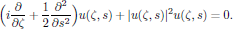(6)

## 5. Mathematical equivalence between temporal and spatial solitons

As seen in the above derivation of the normalized forms of the equations governing wave propagation of temporal and spatial solitons, they are described by exactly the same normalized nonlinear Schrödinger equation. The only difference between the two cases are the ways the normalization is being carried out. In the interpretation of the solutions to the nonlinear Schrödinger equation, the s variable could for the temporal solitons be taken as a normalized time variable, while for the spatial solitons, the s variable could instead be taken as a normalized transverse coordinate.

## 6. Soliton solutions

The nonlinear Schrödinger equations given by Eqs. (3) and (6) possess infinitely many solutions, of which only a few are possible to obtain analytically. In the regime where dvg/ > 0 (that is to say, for which β < 0), an exact temporal soliton solution to Eq. (3) is though obtained when the pulse u(ζ,s) has the initial shapewhere N ≥ 1 is an integer number. Depending on the value of N, solitons of different order can be formed, and the so-called "fundamental soliton" is given for N = 1. For higher values of N, the solitons are hence called "higher order solitons".

The first analytical solution to the nonlinear Schrödinger equation is given for N = 1 asThe shape of this fundamental solution is shown in Fig. 8.For higher order solitons, the behaviour is usually not stable with respect to the normalized distance ζ, but rather of an oscillatory nature, as shown in Fig. 10.1 of the handed out material. (Figure 10.1 is copied from Govind P. Agrawal Fiber-Optic Communication systems (Wiley, New York, 1997).) This figure shows the fundamental soliton together with the third order (N = 3) soliton, and one can see that there is a continuous, oscillatory energy transfer in the s-direction of the pulse. (See also Butcher and Cotter's Fig. 7.8 on page 242, where the N = 4 soliton is shown.)

The solutions so far discussed belong to a class called "bright solitons". The reason for using the term "bright soliton" becomes more clear if we consider another type of solutions to the nonlinear Schrödinger equation, namely the "dark" solitons, given as the solutionswith u0 being the normalized amplitude of the continuous-wave background, φ is an internal phase angle in the range $0\le\phi\le\pi/2$, andFor the dark solitons, one makes a distinction between the "black" soliton for φ = 0, which drops down to zero intensity in the middle of the pulse, and the "grey" solitons for φ ≠ 0, which do not drop down to zero. For the black solitons, the solution for φ = 0 takes ths simpler form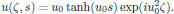The shape of the black fundamental soliton is shown in Fig. 9.Another important difference between the bright and the dark soliton, apart from their obvious difference in appearances, is that the velocity of a dark soliton depends on its amplitude, through the internal phase angle u20ζ. This is not the case for the bright solitons, which propagate with the same velocity irregardless of the amplitude.

The darks soliton is easily pictured as a dark travelling pulse in an otherwise continuous level background intensity. The described dark solitons, however, are equally well applied to spatial solitons as well, for the case n2 > 0, where a dark center of the beam causes a slightly lower refractive index than for the illuminated surroundings, hence generating an effective "anti-waveguide" that compensates for the diffraction experianced by the black center.

## 7. General travelling wave solutions

It should be emphasized that the nonlinear Schr\"odinger equation permits travelling wave solutions as well. On example of such an exact solution is given bywhere n = (1/2)(a2+c2/2). That this in fact is a solution to the nonlinear Schrödinger equation,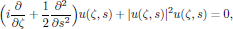(here for simplicity taken for the special case sgn(β) = -1, that is to say for media possessing a positive group velocity dispersion) is straightforward to verify by, for example, using the following Maple blocks:


restart:
assume(s,real);
assume(zeta,real);
assume(a,real);
assume(c,real);
n:=(1/2)*(a\^ 2+c\^ 2/2);
u(zeta,s):=a*sech(a*(s*sqrt(2)-c*zeta)/sqrt(2))
*exp(I*((c/2)*(s*sqrt(2)-c*zeta)+n*zeta));
nlse:=I*diff(u(zeta,s),zeta)+(1/2)*diff(u(zeta,s),s\\$2)
+conjugate(u(zeta,s))*u(zeta,s)\^ 2;
simplify(nlse);



For further information regarding travelling wave solutions and higher order soliton solutions to the nonlinear Schrödinger equation, see P. G. Johnson and R. S. Drazin, Solitons: an introduction (Cambridge University Press, Cambridge, 1989).

## 8. Soliton interactions

One can understand the implications of soliton interaction by solving the NLSE numerically with the input amplitude consisting of a soliton pair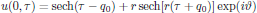with, as previously, sech(x) ≡ 1/\cosh(x), and r is the relative amplitude of the second soliton with respect to the other, θ the phase difference, and 2q0 the initial, normalized separation between the solitons.

A set of computer generated solutions to this pair of initial soliton shapes are shown in the handed-out Fig. 10.6 of Govind P. Agrawal Fiber-Optic Communication systems (Wiley, New York, 1997). In this figure, the upper left graph shows that a pair of solitons may, as a matter of fact, attract each other, forming a soliton pair which oscillate around the center of the moving reference frame.

Another interesting point is that soliton pairs may be formed by spatial solitons as well. In Fig. 9 of the handed-out material, the self-trapping of two spatial solitons, launched with initial trajectories that do not lie in the same plane, are shown. In this experiment, carried out by Mitchell et al. at Princeton , the two solitons start spiraling around each other in a helix, experiencing attractive forces that together with the orbital momentum carried by the pulses form a stable configuration.

## 9. Dependence on initial conditions

In a real situation, one might ask oneself how sensitive the forming of solitons is, depending on perturbations on the preferred sech(s) initial shape. In a real situation, for example, we will rarely be able to construct the exact pulse form required for launching a pulse that will possess the soliton properties already from the beginning.

As a matter of fact, the soliton formation process accepts quite a broad range of initial pulse shapes, and as long as the initial intensity is sufficiently well matched to the energy content of the propagating soliton, the generated soliton is remarkable stable against perurbations. In a functional theoretical analogy, we may call this the soliton "acceptance angle" of initial functions that will be accepted for soliton formation in a medium.

In order to illustrate the soliton formation, one may study Figs. 10.2 and 10.3 of Govind P. Agrawal Fiber-Optic Communication systems (Wiley, New York, 1997)  In Fig. 10.2, the input pulse shape is a Gaussian, rather than the natural sech(s) initial shape. As can be seen in the figure, the pulse shape gradually change towards the fundamental soliton, even though the Gaussian shape is a quite bad approximation to the final sech(s) form.

The forming of the soliton does not only depend on the initial shape of the pulse, but also on the peak intensity of the pulse. In Fig. 10.3, an ideal sech(s) pulse shape, though with a 20 percent higher pulse amplitude than the ideal one of unity, is used as input. In this case the pulse slightly oscillate in amplitude during the propagation, but finally approaching the fundamental soliton solution.

Finally, as being an example of an even worse approximation to the sech(s) shape, a square input pulse can also generate solitons, as shown in the handed-out Fig. 16 of Beam Shaping and Control with Nonlinear Optics, Edited by F. Kajzar and R. Reinisch (Plenum Press, New York, 1998).

 Please note that there is a printing error in Butcher and Cotter's book in the section that deals with the normalization of the nonlinear Schrödinger equation. In the first line of Eq. (7.55), there is an ambiguity of the denominator, as well as an erroneous dispersion term, and the equationshould be replaced by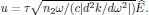(The other lines of Eq. (7.55) in Butcher and Cotter are correct.)

 Please note that there is a printing error in Butcher and Cotter's The Elements of Nonlinear Optics in their expression for this solution, on page 241, row 30, where their erroneous equation "u(ζ,s) = sech(s)exp(-iζ/2)" should be replaced by the proper one, without the minus sign in the exponential.

 M. Mitchell, Z. Chen, M. Shih, and M. Sageev, Self-Trapping of Partially Spatially Incoherent Light, Phys. Rev. Lett. 77, 490 (1996).
DOI: 10.1103/PhysRevLett.77.490

 Exactly the same pictures can be found in Govind P. Agrawal Nonlinear Fiber Optics (Academic Press, New York, 1989).